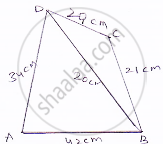Share
Notifications

View all notifications
Books Shortlist
Your shortlist is empty

# Find the Area of a Quadrilateral Abcd in Which Ab = 42 Cm, Bc = 21 Cm, Cd = 29 Cm, Da =34 Cm and Diagonal Bd =20 Cm. - CBSE Class 9 - Mathematics

Login
Create free account

Forgot password?
ConceptArea of a Triangle by Heron’S Formula

#### Question

Find the area of a quadrilateral ABCD in which AB = 42 cm, BC = 21 cm, CD = 29 cm, DA =34 cm and diagonal BD =20 cm.

#### Solution

Given that
Sides of a quadrilateral are AB = 42 cm, BC = 21 cm, CD = 29 cm
DA = 34 cm and diagonal BD = 20 cm
Area of quadrilateral = area of ΔADB + area of ΔBCD.
Now, area of ΔABD
Perimeter of ΔABD
We know that⇒ S = 1/2(𝐴𝐵+𝐵𝐷+𝐷𝐴)
= 1/2(34+42+20)=96
=96/2
= 48 𝑐𝑚

Area of ΔABD = sqrt(S(S-AB)(S-BD)(S-DA))

=sqrt(48(48-42)(48-20)(48-34))

=sqrt(48(14)(6)(28)

=336cm^2

Also for area of ΔBCD,
Perimeter of ΔBCD

2s = BC + CD + BD

⇒S=1/2(29+21+20)+35cm

By using heron’s formulae
Area of ΔBCD =sqrt(s(s-bc)(s-cd)(s-db))

=sqrt(35(35-21)(53-29)(35-20))

=sqrt(210xx210)cm^2

= 210cm^2

∴ Area of quadrilateral ABCD = 336 + 210 = 546 cm^2

Is there an error in this question or solution?

#### APPEARS IN

Solution Find the Area of a Quadrilateral Abcd in Which Ab = 42 Cm, Bc = 21 Cm, Cd = 29 Cm, Da =34 Cm and Diagonal Bd =20 Cm. Concept: Area of a Triangle by Heron’S Formula.
S